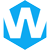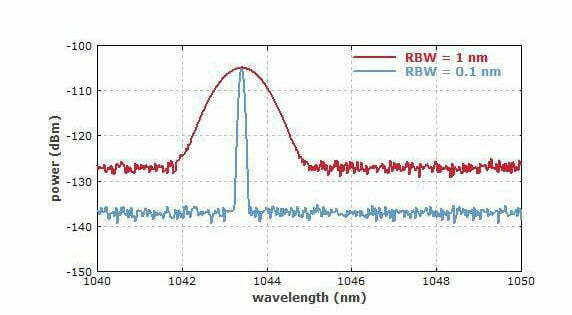# What is signal-to-noise ratio (SNR)?## What is signal to noise ratio?

The signal-to-noise ratio (SNR) is a measure used in science and engineering that compares the level of a desired signal to the level of background noise. It is defined as the ratio of signal power to the noise power. A ratio higher than 1:1 indicates more signal than noise.

## How to calculate signal to noise ratio?

The SNR is usually expressed in decibels (dB). The signal to noise ratio formula is:

SNR=10lg(Ps/Pn)
or
SNR=20lg(Vs/Vn)

Among them, “Ps” and “Pn” represent the effective power of signal and noise respectively, and “Vs” and “Vn” are the effective values of signal and noise voltage.

### Simulation calculation

The following case will use the power ratio as an example to carry out simulation calculations:

```				```
%% Calculating the SNR Simulation
clear; clc; close all; warning off;

% parameter settings
T = 2; % Simulation time
fs = 1000; % Sampling frequency
t = 0 : 1/fs : T;
L = length(t);
A = 10; % Amplitude
fc = 10; % carrier frequency
P_n = 2; % noise power

% generate simulated signal
signal = A * cos(2 * pi * fc .* t); % generate a valid signal
noise = sqrt(P_n) .* randn(1, L); % Generate white Gaussian noise signal

% Calculating SNR
P_s = sum(signal .^ 2) / L; % signal power
P_n = sum(noise .^ 2) / L; % noise power
SNR = 10 * log10(P_s / P_n); % signal-to-noise ratio
fprintf('\t Ps：%.2fW\n', P_s);
fprintf('\t Pn：%.2fW\n', P_n);
fprintf('\t SNR：%.2fdB\n', SNR);

figure(1); clf;
subplot(2, 1, 1);
plot(t, signal, 'b', 'linewidth', 2); hold on;
plot(t, noise, 'k', 'linewidth', 1); hold on;
plot(t, signal+noise, 'g', 'linewidth', 1);
xlabel('t'); ylabel('y'); axis('tight'); title('All waveforms'); set(gca, 'fontsize', 14);
subplot(2, 1, 2);
plot(t(100:500), signal(100:500), 'b', 'linewidth', 2); hold on;
plot(t(100:500), noise(100:500), 'k', 'linewidth', 1); hold on;
plot(t(100:500), signal(100:500)+noise(100:500), 'g', 'linewidth', 1);
legend(['signal power≈', num2str(P_s, '%.2f'), 'W'], ['noise power≈', num2str(P_n, '%.2f'), 'W'], ...
['SNR≈', num2str(SNR, '%.2f'), 'dB'], 'location', 'eastoutside');
xlabel('t'); ylabel('y'); axis('tight'); title('Zoom in to observe signal details'); set(gca, 'fontsize', 14);
set(gcf, 'position', [12, 60, 1450, 650]);
```
```

ADC SNR is used to measure the quality of a digital-to-analog converter (DAC) or analog-to-digital converter (ADC). The higher the ADC SNR value, the better the performance of the converter system. It is essential for any signal system to maintain a good ADC SNR value in order to provide accurate output signals.

SNR=6.02N+1.76dB

“N” is the number of bits of the ADC.

### Formula Derivation

The figure below is a schematic diagram of the quantization noise of an ideal ADC. As can be seen from the figure below, for a linear input analog signal, the ADC will produce a stepped output. The error waveform of this input and output is similar to a sawtooth with peak-to-peak value q=1LSB Wave, its effective value RMS calculation process is shown in formula (1) (q=1LSB), LSB calculation process is shown in formula (2), where FS is the input voltage range of ADC.Since the signal-to-noise ratio is the effective value (RMS) of the signal divided by the effective value (RMS) of the noise, we can get the formula (3):For a full-scale input sinusoidal signal as shown in formula (4), formula (5) can be obtained according to formula (4):For a full-scale ADC, its input range is 0-FS, then the amplitude range of the input sinusoidal signal is 0-Fs/2, see the schematic diagram below, so the denominator in formula (4) is 2.

So far, we know the effective value (RMS) of the signal – formula (5), and the effective value (RMS) of the ADC quantization noise – formula (1). Now we put the formula (5) and formula (1) into formula (3) to get formula (6):After simplifying the formula (6), we can obtain formula 7 as below:#### Share:Aidan Taylor

I am Aidan Taylor and I have over 10 years of experience in the field of PCB Reverse Engineering, PCB design and IC Unlock.

## Recommended Post

### Need Help?

Don't hesitate - Get in touch today to find out how we can help take your project to the next level.

Scroll to Top## Start to Achieve Your PCB Project

Hello 👋
Can we help you? Chat with us on WhatsApp↓, or send an email to us: info@reversepcb.com